International
Tables for
Crystallography
Volume B
Reciprocal space
Edited by U. Shmueli

International Tables for Crystallography (2006). Vol. B, ch. 1.2, p. 10   | 1 | 2 |

## Section 1.2.2. General scattering expression for X-rays

P. Coppensa*

aDepartment of Chemistry, Natural Sciences & Mathematics Complex, State University of New York at Buffalo, Buffalo, New York 14260-3000, USA
Correspondence e-mail: coppens@acsu.buffalo.edu

### 1.2.2. General scattering expression for X-rays

| top | pdf |

The total scattering of X-rays contains both elastic and inelastic components. Within the first-order Born approximation (Born, 1926) it has been treated by several authors (e.g. Waller & Hartree, 1929; Feil, 1977) and is given by the expression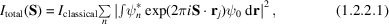where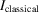is the classical Thomson scattering of an X-ray beam by a free electron, which is equal to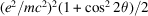for an unpolarized beam of unit intensity, ψ is the n-electron space-wavefunction expressed in the 3n coordinates of the electrons located at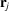and the integration is over the coordinates of all electrons. S is the scattering vector of length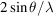.

The coherent elastic component of the scattering, in units of the scattering of a free electron, is given by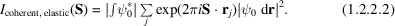If integration is performed over all coordinates but those of the jth electron, one obtains after summation over all electrons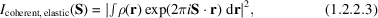whereis the electron distribution. The scattering amplitude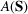is then given by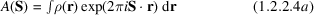or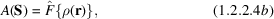where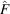is the Fourier transform operator.

### References

Born, M. (1926). Quantenmechanik der Stoszvorgänge. Z. Phys. 38, 803.Google Scholar
Feil, D. (1977). Diffraction physics. Isr. J. Chem. 16, 103–110.Google Scholar
Waller, I. & Hartree, D. R. (1929). Intensity of total scattering X-rays. Proc. R. Soc. London Ser. A, 124, 119–142.Google Scholar